Courses

Test: Numbers - 3

20 Questions MCQ Test Mathematics for Class 3: NCERT | Test: Numbers - 3

Description
Attempt Test: Numbers - 3 | 20 questions in 40 minutes | Mock test for Class 3 preparation | Free important questions MCQ to study Mathematics for Class 3: NCERT for Class 3 Exam | Download free PDF with solutions
QUESTION: 1

Madhu wrote a number on the blackboard as shown below.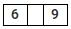If it is formed from three different digits, then which of the following digit could be placed in the gap to make it the greatest number out of the given options?

Solution:

(a) 609
(b) 659
(c) 699
(d) 689
Out of the above numbers 699 is greatest but its all digits are not different. So, 689 is the required greatest number.

QUESTION: 2

Sohan has two baskets full of some number tokens. He likes the numbers that are in red basket. Which of the following numbers formed by using given basket numbers will he like most?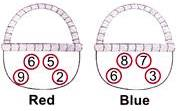Solution:

Since, Sohan likes the numbers in the red basket, so he will like the numbers which are formed from the digits 6, 5, 9 and 2. So, he likes 6529.

QUESTION: 3

Mr Kapoor's house number is having nine at hundreds place. Which could not be his house number?

Solution:

From the given options, only 7896 does not have 9 at hundreds place. So, it cannot be Mr Kapoor's house number.

QUESTION: 4

Mrs Sharma wrote a 2-digit number on the blackboard. Three students Lalit, Vinay and Prashant said the following statements about that number.
Lalit : "It is a 2-digit number but not the least".
Vinay : "It is the predecessor of least 3-digit number".
Prashant : "It is having three different digits".

If only two of the three students said the truth, who is definitely lying?

Solution:

Prashant said that all three digits are different but Mrs Sharma wrote 2-digit number. So, he is definitely lying.

QUESTION: 5

Four boys are playing a number game. Each of them are writing their favourite number. Who wrote the greatest number?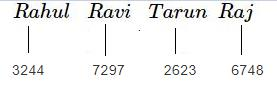Solution:

Out of the given numbers, 7297 is the greatest. So, Ravi wrote the greatest number.

QUESTION: 6

The given chart shows the number of eggs produced on a farm in the first five months of the year.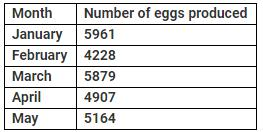Solution:

The number of eggs produced in May were less than in March is true because, 5164 < 5879.

QUESTION: 7

If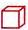represents 1, then write the number represented by the given diagram.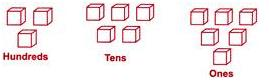Solution:

Hundreds = 3 Tens = 5 Ones = 6 So, the number is 356.

QUESTION: 8

Match the following: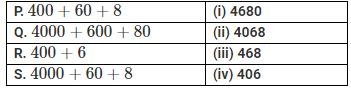Solution:

P. 400+60+8=468
Q. 4000+600+80=4680
R. 400+6=406
S. 4000+60+8=4068
So, P→(iii), Q→(i), R→ (iv), S→(ii)

QUESTION: 9

Identify the number using the given clues.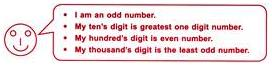Solution:

The given number is an odd number, so the one's digit will be odd. The ten's digit is highest one digit number i.e. 9. Hundred's digit is even number and thousands digit is the least odd number i.e. 1.
So, from the given options the number is 1495.

QUESTION: 10

Sonali spilled ink drops on her homework sheet as shown below.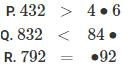Which digits must be written to make the above sentences CORRECT?

Solution:

P. 432 > 426
Q. 843 < 848
R. 792 = 792
So, option (a) is correct.

QUESTION: 11

Which even number will come right before 28?

Solution:
QUESTION: 12

Which one among the following is an odd number less than 29 and greater than 19?

Solution:
QUESTION: 13

Which among the following is not a composite number?

Solution:
QUESTION: 14

Find the number which is not a factor of 3 and 4 both?

Solution:
QUESTION: 15

Which among the following is incorrect pain of twin primes?

Solution:
QUESTION: 16

Find the difference of the place of digit 2 and face value of 9 digits 9 in the number 72398.

Solution:
QUESTION: 17

Find the predecessor of 37 + 2 - 3.

Solution:
QUESTION: 18

What should be subtracted from 13 + 21 + 9 to get the successor of 22?

Solution:
QUESTION: 19

Arrange the following number in descending order: 21, 29, 18, 32, 26

Solution:
QUESTION: 20

Which among the following is incorrect for the number 8749?

Solution:Use Code STAYHOME200 and get INR 200 additional OFF Use Coupon Code

Track your progress, build streaks, highlight & save important lessons and more!

Similar ContentRelated tests